# RRB ALP 2018 Practice Test Papers | Reasoning Questions (Day-41)

Dear Aspirants, Here we have given the Important RRB ALP & Technicians Exam 2018 Practice Test Papers. Candidates those who are preparing for RRB ALP 2018 can practice these Reasoning Questions to get more confidence to Crack RRB 2018 Examination.

[WpProQuiz 2186]

Click “Start Quiz” to attend these Questions and view Solutions

1. If the words are organised in reverse order of what they appear in dictionary, which word will come in the third place.

(a) Occulist

(b) Odium

(c) Odious

(d) Ordeum

1. In a certain code, DOWNBEAT is written a TABEWNDO. How will the word PROSPECT be written in that code?

(a) TCEPSORP

(b) TCPEOSPR

(c) TCPESOPR

(d) TCPEOSRP

1. Suket has three daughters and each daughter has a brother. How many male members are there in the family?

(a) 4

(b) 2

(c) 3

(d) 1

1. If South East becomes North, then what will South West become?

(a) North

(b) West

(c) East

(d) North West

1. In the following question, select the number which can be placed at the sign of question mark (?) from the given alternatives.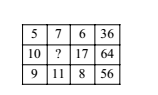(a) 5

(b) 6

(c) 7

(d) 8

1. After interchanging ÷ and +, 12 and 18, which one of the following equations becomes correct?

(a) (90 × 18) + 18 = 60

(b) (18 + 6) ÷ 12 = 2

(c) (72 ÷ 18) × 18 = 72

(d) (12 + 6) × 18 = 36

1. Priyank ranks 6th from the bottom and 28th from the top in a class. How many students are there in the class?

(a) 31

(b) 32

(c) 33

(d) 34

1. If PAINT is coded as 74128 and EXCEL is coded as 93596, how is ACCEPT coded?

(a) 459578

(b) 457958

(c) 459758

(d) 455978

1. Choose the correct figure that represents the given relation:

Blue eyes, females, doctors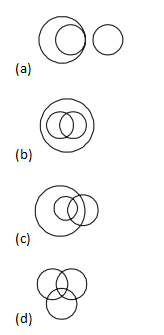1. From the given alternative words, select the word which cannot be formed using the letters of the given word:

TRIVANDRUM

(a) RAIN

(b) DRUM

(c) TRAIN

(d) DRUK

1. Answer: (c)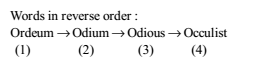2. Answer: (b)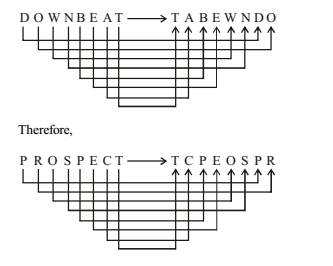3. Answer: (b)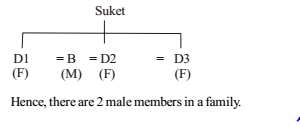If South East becomes North then south west becomes east as shown in direction chart.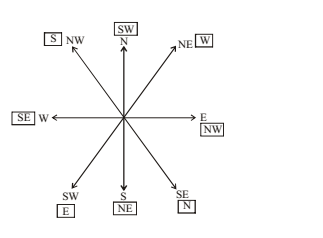According to question, As, (5 + 7 + 6) × 2 = 36

(9 + 11 + 8) × 2 = 56

Similarly, (10 +? + 17) × 2 = 64

(10 +? + 17) =64/2= 32

27 +? = 32

? = 32 – 27 = 5

(12 + 6) × 18 = 36 => (18 ¸ 6) × 12 = 36 => 3 × 12 = 36

Priyank is 6th from the bottom 28th from top. So, total number of students = 6 + 28 – 1 = 33

1. Answer: (d)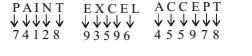Some blue eyed may be females and vice-versa. Some females may be doctors and vice-versa. Some blue eyed. may be doctors and vice-versa. Some blue eyed females may be doctors.